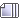###Author Topic: GreenBH - Total idiot, big ego cunt  (Read 20054 times)

#### dom0

unless you got something extra ;)

#### thegoodperry

It's interesting how dom0's posts read like a piece of abstract literature they force you to read in 10th grade English. Like every sentence is just a new formula of confusion and just when you think you understand roughly what it's saying you get hit by another curveball stanza. And that stuffs so obscure that not even sparknotes covers it, so you're just struggling to paraphrase each line but to no avail

#### GreenBH³

unless you got something extra ;)

Take a shot each time he posts with a winky face.

#### dom0

I give you a sentence, you give me a paragraph.

might i ask what this has to do with anything? its better expression. sure you have to read but who hates that eh? your bleeding me dry here. propane tanks dont fuel themselves you know.

#### Verification

Defense of the Autism 2literally how can this dude make me this confused when he natively speak english. autistic people are not even this bad at forming setences, and they have actual disabilities. this dude probably is on the low end of the spectrum or something because every post is like being smacked in the face out of nowhere but from a different angle every time

#### Grimlock²

nah my nigeria certified scanner is picking up denser levels of autism from this account. I've never seen such aggressive readings before. Whoever he is, he's definitely stronger than blockchip
High Betelgeuse when he planned and executed the first forum bombing is the only thing in the database close. Its Master Matthew X prime Maxx levels

#### dom0

well somebody's bound to die and i'm under aged so anybody up for it ;) ;) ;) ;) ;) ;) ;) ;)

#### dom0

;) ;) ;) ;) ;) ;) ;) ;) ;) ;) ;) ;) ;) ;) ;) ;) ;) ;) ;) ;) ;) ;) ;) ;) ;) ;) ;) ;) ;) ;) ;) ;) ;) ;) ;) ;) ;) ;) ;) ;) ;) ;) ;) ;) ;) ;) ;) ;) ;) ;) ;) ;) ;) ;) ;) ;) ;) ;) ;) ;) ;) ;) ;) ;) ;) ;) ;) ;) ;) ;) ;) ;) ;) ;) ;) ;) ;) ;) ;) ;) ;) ;) ;) ;) ;) ;) ;) ;) ;) ;) ;) ;) ;) ;) ;) ;) ;) ;) ;) ;) ;) ;) ;) ;) graves dont fill themselves also ;) ;) ;) ;) ;) ;) ;) ;) ;) ;) ;) ;) ;) ;) ;) ;) ;) ;) ;) ;) ;) ;) ;) ;) ;) ;) ;) ;) ;) ;) ;) ;)

#### Verification

well somebody's bound to die and i'm under aged so anybody up for it ;) ;) ;) ;) ;) ;) ;) ;)
When the subject matter doesn't come from the topic, you like to respond, eh? You seem promiscuously ready to incapacitiate yourself, sir. Well, i'll have you understand that i'm ready for it. And I am preparing to come over.

#### TheABELBOTO

oh stuff he's going nuclear guys

#### datiel12

well somebody's bound to die and i'm under aged so anybody up for it ;) ;) ;) ;) ;) ;) ;) ;)
hey forgetlord mind not derailing the topic and mind not acting like a complete total retard

#### Grimlock²

well somebody's bound to die and i'm under aged so anybody up for it ;) ;) ;) ;) ;) ;) ;) ;)
Autism unleashed. Literally

;) ;) ;) ;) ;) ;) ;) ;) ;) ;) ;) ;) ;) ;) ;) ;) ;) ;) ;) ;) ;) ;) ;) ;) ;) ;) ;) ;) ;) ;) ;) ;) ;) ;) ;) ;) ;) ;) ;) ;) ;) ;) ;) ;) ;) ;) ;) ;) ;) ;) ;) ;) ;) ;) ;) ;) ;) ;) ;) ;) ;) ;) ;) ;) ;) ;) ;) ;) ;) ;) ;) ;) ;) ;) ;) ;) ;) ;) ;) ;) ;) ;) ;) ;) ;) ;) ;) ;) ;) ;) ;) ;) ;) ;) ;) ;) ;) ;) ;) ;) ;) ;) ;) ;) graves dont fill themselves also ;) ;) ;) ;) ;) ;) ;) ;) ;) ;) ;) ;) ;) ;) ;) ;) ;) ;) ;) ;) ;) ;) ;) ;) ;) ;) ;) ;) ;) ;) ;) ;)
:egg:
Unova stop loving posting let the autism busters me phantos n greenbh handle this

#### dom0

When the subject matter doesn't come from the topic, you like to respond, eh? You seem promiscuously ready to incapacitiate yourself, sir. Well, i'll have you understand that i'm ready for it. And I am preparing to come over.

what are you packing. gin, vodka, fancy wine~, or just hard ass beer from the local bar.

#### Grimlock²

what are you packing. gin, vodka, fancy wine~, or just hard ass beer from the local bar.
Ever tried everclear? You should chug a handle, youd be doing the world a favor

#### dom0

hey forgetlord mind not derailing the topic and mind not acting like a complete total retard

why does everybody thrown mentally disabled terms at everyone. here's a tip i think i need it myself so i can leave this dumb stuff. G R O W   U P!!